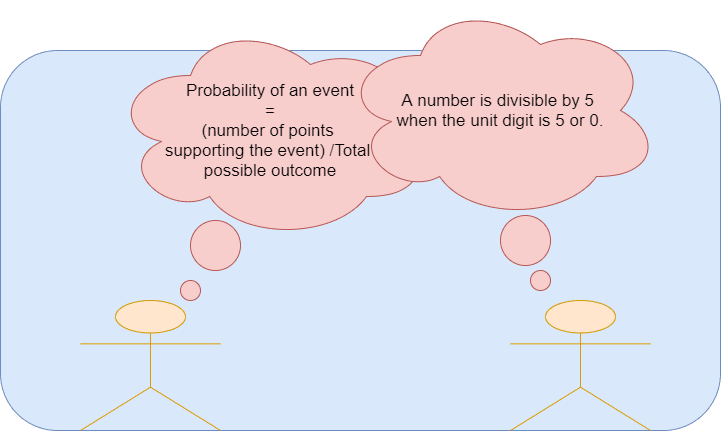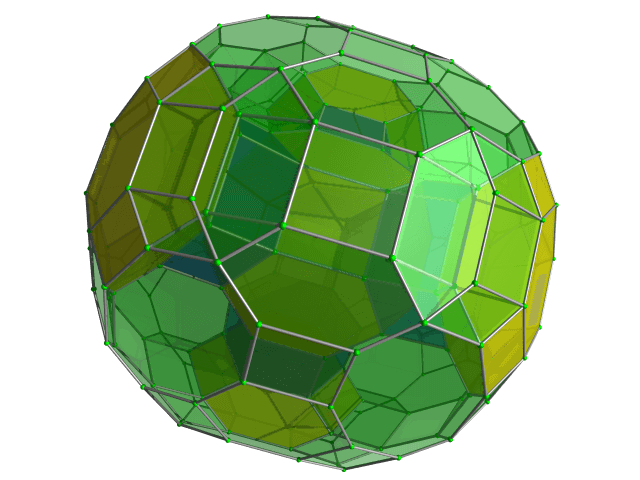Categories

# Probability of an event- AMC 8 2009 Problem 13

Try this beautiful problem from AMC 8. It involves probability and divisibility. We provide sequential hints so that you can try the problem.

## Competency in Focus: Probability of an event

This problem from American Mathematics Contest 8 (AMC 8, 2019) is based on calculation of probability of an event using the concept of divisibility. It is Question no. 13 of the AMC 8 2009 Problem series.

## First look at the knowledge graph:-## Next understand the problem

A three-digit integer contains one of each of the digits $1$, $3$, and $5$. What is the probability that the integer is divisible by $5$? $\textbf{(A)}\ \frac{1}{6} \qquad \textbf{(B)}\ \frac{1}{3} \qquad \textbf{(C)}\ \frac{1}{2} \qquad \textbf{(D)}\ \frac{2}{3} \qquad \textbf{(E)}\ \frac{5}{6}$
##### Source of the problem

American Mathematical Contest 2009, AMC 8 Problem 13

### Finding probability of an event using the concept of divisibility

5/10
##### Suggested Book
Challenges and Thrills in Pre College Mathematics Excursion Of Mathematics

Do you really need a hint? Try it first!
First note that a number is divisible by $5$ if the unit digit is $5$ or $0$
Can you find all the three digit numbers that can be made using the digits given
So the three digit numbers are $135,153,351,315,513,531$. among these only two numbers are divisible by $5$
Probability of an event = $\frac{\textbf{Number points supporting the event}}{Total number of outcomes}$ Ans=$\frac{\textbf{Number of 3 digit numbers divisible by 5}}{\textbf{Number of 3 digits number can be made}}=\frac{2}{6}=\frac{1}{3}$

## AMC - AIME Program

AMC - AIME - USAMO Boot Camp for brilliant students. Use our exclusive one-on-one plus group class system to prepare for Math Olympiad## External Tangent | AMC 10A, 2018 | Problem 15

Try this beautiful Problem on geometry based on circle from AMC 10A, 2018. Problem-15. You may use sequential hints to solve the problem.

## Dice Problem | AMC 10A, 2014| Problem No 17

Try this beautiful Problem on Probability from AMC 10A, 2014. Problem-17, You may use sequential hints to solve the problem.

## Problem on Curve | AMC 10A, 2018 | Problem 21

Try this beautiful Problem on Co-ordinate geometry from AMC 10A, 2018. Problem-21, You may use sequential hints to solve the problem.

## Right-angled Triangle | AMC 10A, 2018 | Problem No 16

Try this beautiful Problem on triangle from AMC 10A, 2018. Problem-16. You may use sequential hints to solve the problem.

## Finding Greatest Integer | AMC 10A, 2018 | Problem No 14

Try this beautiful Problem on Algebra from AMC 10A, 2018. Problem-14, You may use sequential hints to solve the problem.

## Length of the crease | AMC 10A, 2018 | Problem No 13

Try this beautiful Problem on triangle from AMC 10A, 2018. Problem-13. You may use sequential hints to solve the problem.

## Right-angled shaped field | AMC 10A, 2018 | Problem No 23

Try this beautiful Problem on triangle from AMC 10A, 2018. Problem-23. You may use sequential hints to solve the problem.

## Area of region | AMC 10B, 2016| Problem No 21

Try this beautiful Problem on Geometry on Circle from AMC 10B, 2016. Problem-20. You may use sequential hints to solve the problem.

## Coin Toss Problem | AMC 10A, 2017| Problem No 18

Try this beautiful Problem on Probability from AMC 10A, 2017. Problem-18, You may use sequential hints to solve the problem.

## GCF & Rectangle | AMC 10A, 2016| Problem No 19

Try this beautiful Problem on Geometry on Rectangle from AMC 10A, 2010. Problem-19. You may use sequential hints to solve the problem.

This site uses Akismet to reduce spam. Learn how your comment data is processed.